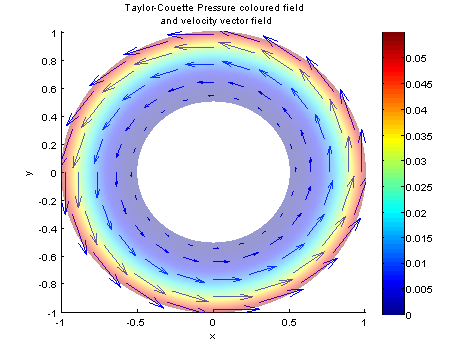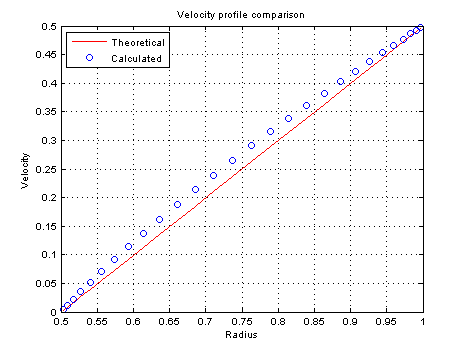# Simulation of the Taylor-Couette flow

Coded by Paul Valcke and Luis Bernardos. This codes shows the simulation of a flow around a cylinder. We solved this problem using the steady Navier-Stokes equations. Velocity-pressure non-linear coupling is solved by means of a Newton method, based on the Jacobian matrix. In this code we use a first-order march in time for integrating through time.

There exist an analytical solution for this flow, then we compare it with theory in the last section of this page.

# Preliminary thoughts about the flow

The Taylor-Couette flow consists on two concentrical cylinders. The outter-most cylinder rotates at constant speed, adding a rotational velocity to the interior flow by means of the viscosity. This phenomena is used for measuring the viscosity of fluids.

Through this code we want to perform a calculation of the airflow taking into consieration that we want to use a “deformed” circular mesh, and we want to solve the cartesian-Steady Navier-Stokes equations:

\displaystyle u_j\partial_ju_i = -\partial_ip + \frac{1}{Re}\partial_{jj}^2u_i

Ultimately, we want to compare the velocity profile obtained with the theoretical one.

clear all; clf;


# Parameters

Here the user can change the geometry and physics of the flow.

%%%% parameters
Re=10; % reynolds number
Rmax = 1; % Outter cylinder radius
Rmin = 0.5; % Inner cylinde radius
Ntheta=101; % number of grid nodes in theta
Nrho=30; %number of grid nodes in rho
pts=5; % number of points in finite difference stencils
alpha=1; % Under-relaxation
maxIter = 200;
resSTOP = 1e-5;

Lx = 2*pi*Rmax; Lx_vector = linspace(0,2*pi,Ntheta);
Ly = Rmax-Rmin;

% differentiation
[d.x,d.xx,d.wx,x]=dif1D('fd',0,1,Ntheta,pts);
[d.y,d.yy,d.wy,y]=dif1D('fd',0,1,Nrho,pts);
[D,l,~,~,Z,I,NN]=dif2D(d,x,y);


# Mesh

We make use of Lspacing for shrinking the mesh near the walls. Also, we make use of map2D for transforming the differentation matrices.

% Meshing
[Theta, Rho] = meshgrid(linspace(0,2*pi,Ntheta),Rmin + Lspacing(Nrho,1,Rmax-Rmin));
[X, Y] = pol2cart(Theta,Rho);
D=map2D(X,Y,D);
D.lap=D.yy+D.xx;

u=(1:NN)'; v=u+NN; p=v+NN;
II=speye(3*NN);
DD=blkdiag(D.y,D.y,D.y);


# Boundary Conditions

The boundary conditions are all-dirichlet. No input nor output flow for this case. However, as the mesh joins itself at the overlapping boundary, a link condition has to be established, in both the continuity of u, v and p as well as the derivatives normal to the overlapping boundary (tangency of the unknowns).

% Boundary Conditions
dir=[l.top;l.bot]; % where to put Dirichlet on u and v
lnk1=[u(l.left); v(l.left); p(l.left)];
lnk2=[u(l.right); v(l.right); p(l.right)];
p0loc=1;
loc=[u(dir); v(dir); p(p0loc); ... % Dirichlet

C=[II([u(dir);v(dir);p(p0loc)],:);% Dirichlet on u,v at walls
II(lnk1,:)-II(lnk2,:); % Continuity of the value
DD(lnk1,:)-DD(lnk2,:)]; % Continuity of the normal derivative


We compute a suitably initial guess that will easen the convergence and reduce the number of iterations.

% initial guess
Ur=Rho-Rmin; % Modulus of the tangent velocity imposed on the outter cylinder.
U=-sin(Theta).*Ur;
V=cos(Theta).*Ur;
P=0*X(:);

q0=[U(:);V(:);P(:)];


# Calculation

% % STEADY
% Newton iterations
disp('Newton loop')
q=q0;
quit=0;count=0;
while ~quit

% the present solution and its derivatives
U=q(u);
V=q(v);
P=q(p);
Ux=D.x*U; Uy=D.y*U;
Vx=D.x*V; Vy=D.y*V;
Px=D.x*P; Py=D.y*P;

% nonlinear function
f=[-(U.*Ux+V.*Uy)+(D.lap*U)/Re-Px; ...
-(U.*Vx+V.*Vy)+(D.lap*V)/Re-Py; ...
D.x*U+D.y*V];

% Jacobian
A=[-( spd(Ux) + spd(U)*D.x + spd(V)*D.y )+(D.lap)/Re, -spd(Uy), -D.x; ...
-spd(Vx),  -(spd(Vy) + spd(V)*D.y + spd(U)*D.x )+(D.lap)/Re, -D.y; ...
D.x, D.y, Z];

% Boundary conditions
f(loc)=C*(q-q0);
A(loc,:)=C;
The following commented lines are used for determining whether the matrix A is 

inversible or not. If the solution of Agives a NaN, then it can be beause A is not inversible.

%     % checking the null space of A
%     [UU,S]=eig(full(A));
%    s=diag(S);  [t,o]=sort(-real(s));
%    s=s(o); UU=UU(:,o);
%    rem=abs(s)>1000; s(rem)=[]; UU(:,rem)=[];
%
%    sel=abs(s)<1e-5;
%    s=s(sel), UU=UU(:,sel);
%
%    for num=1:length(s);
%    subplot(3,3,num)
%        U=UU(u,num);
%    V=UU(v,num);
%    P=UU(p,num);
%
%    nu=norm(U)
%    nv=norm(V)
%
%    surf(X,Y,reshape(abs(P),Nrho,Ntheta)); view(2); hold on
%      quiver(X,Y,reshape(U,Nrho,Ntheta),reshape(V,Nrho,Ntheta));
%    end
%
%
%     break

% plotting
Module = sqrt((U.^2+V.^2));
hold on
uu=reshape(U,Nrho,Ntheta); vv=reshape(V,Nrho,Ntheta);
quiver(X(1:5:end,1:5:end),Y(1:5:end,1:5:end),uu(1:5:end,1:5:end),vv(1:5:end,1:5:end));
xlabel('x'); ylabel('y'); title(sprintf('Taylor-Couette Pressure coloured field\nand velocity vector field')); grid off;hold off
axis([-1,1,-1,1]*Rmax)
hold off
drawnow

% convergence test
res=norm(f);
disp([num2str(count) '  ' num2str(res)]);
if (count>maxIter) || (res>1e5); disp('no convergence');break; end
if res<resSTOP; quit=1; disp('converged'); continue; end

% Newton step
q=q-A\f;
count=count+1;
end

colorbar;
set(gcf,'paperpositionmode','auto')
print('-dpng','-r80',sprintf('TaylorCouette.png'));


# Results and ValidationOverall velocity representation.

The Taylor-Couette flow phisically has a linear velocity profile between the inner cylinder and the outter cylinder. Therefore, the velocity profile will be V_{th} = -R_{min} + \frac{U_{rot}}{R_{max}-R_{min}}\rho. Excellent agreement between theory and calculations is reached:Comparison of velocity profiles.

x_plot = X(l.left);
V_theory = -Rmin + (max(max(Ur))/(Rmax-Rmin)).*x_plot;
figure;
plot(x_plot,V_theory,'-r','DisplayName','Theoretical'); hold on
plot(x_plot,V(l.left),'ob','DisplayName','Calculated'); hold off
grid on;